# Time Difference

Calculating the difference between two times in Excel can be tricky. Times are handled internally as numbers between 0 and 1.

### Simple Formula

Let's start with a simple formula that calculates the difference between two times in the same day.

1. Simply subtract the start time from the end time.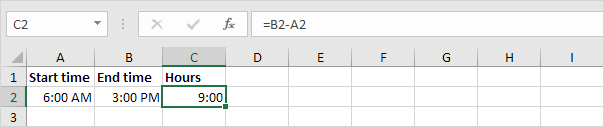Note: AM is used for times in the night and morning. PM is used for times in the afternoon and evening. Change the Time format to display a time with or without AM/PM.

2. Change the number format of the values in cell A2, B2 and C2 to General.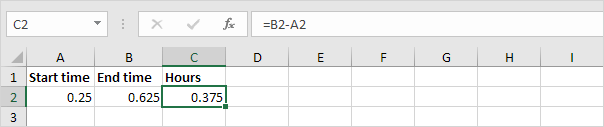Note: times are handled internally as numbers between 0 and 1. For example, 6:00 AM is represented as 0.25 (quarter through the day).

### Time Difference Formula

The simple formula shown above doesn't work if the start time is before midnight and the end time is after midnight.

1. Times that are negative show as ######.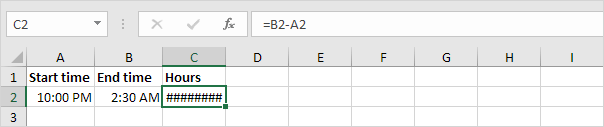2. To clearly see this, change the number format of the values in cell A2, B2 and C2 to General.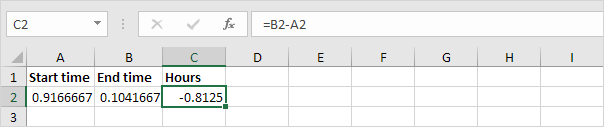3. The time difference formula below always works.Explanation: if the end time is greater than or equal to the start time, simply subtract the start time from the end time. If not, add 1 (full day) to the end time to represent a time on the next day and subtract the start time.

### Time Difference in Hours as Decimal Value

To calculate the difference between two times in hours as a decimal value, multiply the previous formula by 24 and change the number format to General.

1. The formula below does the trick.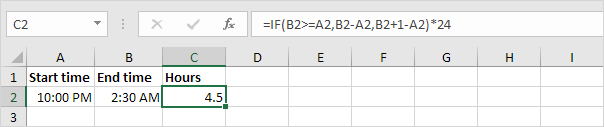### Time Sheet

Calculate total hours, regular hours and overtime hours with our timesheet calculator.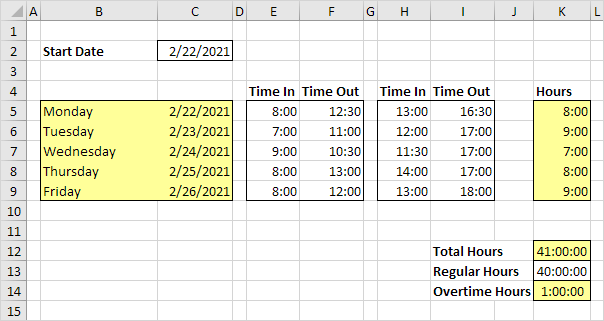Go to Next Chapter: Text Functions